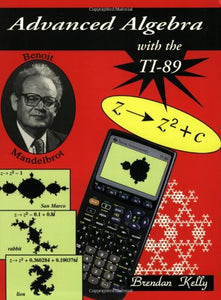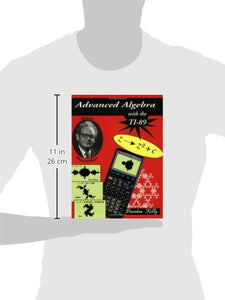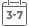# Advanced Algebra With The Ti-89

Vendor
Brendan Kelly Pub
Regular price
\$38.21
Sale price
\$28.89
Quantity must be 1 or more

Attention: For textbook, access codes and supplements are not guaranteed with used items.This exciting book contains 20 instructional lessons that guide students step-by-step through sequences of activities that help them learn the mathematical concepts using the TI-89 graphing calculator. Keying sequences are provided for each lesson. Students are given lots of exercises for practice of the concepts and complete solutions are given for most of the exercises.Topics included are:

Fundamental Theorem of Algebra

polynomial functions

polynomial equations

rational functions trigonometric functions

exponential functions

logarithmic functions

linear systems

linear programming

matrices

congruence arithmetic

complex numbers

fractals